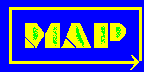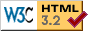# Materials Algorithms ProjectProgram Library

## Program MAP_CRYSTAL_PROGRAM

### Provenance of Source Code

Phase Transformations Group,
Department of Materials Science and Metallurgy,
University of Cambridge,
Cambridge, U.K.

Top | Next

### Purpose

An interactive program for the analysis of crystal structures. crystal.f will require subroutines available in this library to be added. crystal.for is the complete program, ready for compilation.

Top | Next | Prev

### Specification

 Language: FORTRAN Product form: Source code

This is a self-contained program.

Top | Next | Prev

### Description

The program gives guidance to the user when choices have to be made. The initial choice is selecting which of the following are to be performed:-

1. Analysis of Electron Diffraction

The camera constant may be known, in which case the program needs an input of two d-spacings from a pair of reciprocal lattice vectors and the angle between the two vectors concerned. The crystal system and lattice type must also be specified.

It is often the case that the camera constant is unknown. The program then operates without d-spacings, using instead an input of the ratio of the lengths of two reciprocal lattice vectors, as measured from the diffraction pattern. If the crystal type is unknown then it can be assumed to be primitive in the first instance. Crystallographically equivalent solutions can be avoided, but this may increase the computing time required.

2. Calculation of Metric Tensor

This calculates the metric tensor, which is a coordinate transformation relating the real and reciprocal axes.

3. Interplanar Spacings

This calculates the spacing of planes in crystals of arbitrary system, taking account of absences due to lattice types.

4. Angles between Vectors

This calculates the angles between two vectors in an arbitrary crystal system, whether the vectors are plane normals (i.e. reciprocal vectors) or directions (i.e. real vectors). Any combination of these two kinds of vectors may be used as the input.

5. Convert between Real and Reciprocal Space

This converts the components of a vector from real to reciprocal space, or vice versa, for any crystal system. It can be used to identify a plane normal which is parallel to a specified direction, or a direction which is parallel to a specified plane normal.

6. Axis-Angle Pairs

This calculates axis-angle pairs relating crystals belonging to the cubic system. It also calculates rotation matrices and symmetry-related descriptions.

7. Orientation Relations

This calculates the orientation relationship between two crystals, each of which may belong to any crystal system, from a knowledge of a pair of vectors (in any combination of real or reciprocal) from each crystal. For example,
(1 1 0)A | | (1 1 1)B and [1 0 0]A | | [-1 0 1]B.

It calculates coordinate transformation matrices, generates lists of planes (and directions) which are parallel in the two crystals, and allows chosen vectors to be examined in detail.

8. Documentation

Gives information on the calculations performed by the program.

9. Four Index Notation

This converts four index notation to three index notation, and vice versa. Miller-Bravais notation is used for plane normals and Weber notation for directions, allowing correct use of the Weiss zone law.

10. Vector Size

This calculates the vector magnitude in Ångstroms.

11. Exit Program

Top | Next | Prev

### References

1. H.K.D.H. Bhadeshia, The Geometry of Crystals, Institute of Metals, London, 1987.
2. H.K.D.H. Bhadeshia, Chapter on crystallography in Microstructural Characterisation of High Temperature Materials, ed. E. Metcalfe, Institute of Metals, London, 1988.

Top | Next | Prev

### Input parameters

N/A - complete program

### Output parameters

All output is to STDOUT

Top | Next | Prev

### Error Indicators

None.

Top | Next | Prev

### Accuracy

No information supplied

Top | Next | Prev

None.

Top | Next | Prev

### Example

#### 1. Program text

```       Complete program

```

#### 2. Program data

```None supplied.

```

#### 3. Program results

```None supplied.

```

Top | Next | Prev

### Auxiliary Routines

The subroutines called by this program correspond to non-interactive routines described elsewhere in the documentation :-

Top | Next | Prev

### Keywords

electron diffraction, metric tensor, angle, real and reciprocal space, axis-angle pair, four index notation

Top | Next | Prev

Top | Index | MAP Homepage